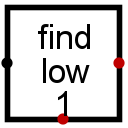#Bit Finder

 Library: Arithmetic Introduced: 2.6.1 Appearance:## Behavior

The component takes a multi-bit input and determines the the index of a bit, where the index is computed by counting from 0 as the lowest-order bit. Exactly which index it computes depends on the Type attribute, as illustrated by the examples in the below table for the 8-bit sample input 11010100.

Type Output for 11010100
Lowest-order 1 2
Highest-order 1 7
Lowest-order 0 0
Highest-order 0 5

For the lowest-order 1, the output is 2 because if you index the bits starting from 0 for the lowest-order bit, the first 1 you will find is at index 2. (The bits at indices 0 and 1 are both 0.) For the highest-order 1, the output is 7 because the topmost 1 bit is at index 7 (again counting from the lowest-order bit as 0).

The component's output on the south edge indicates whether the desired bit was found at all. In the above examples involving the input 11010100, the south output is 1 in all cases. But if the input were 00000000 and the component is to find the lowest-order 1, then the south output would be 0 — and the output on the east edge would be 0 as well.

If while searching for the desired value, a value that is neither 0 or 1 is found (the bit could be floating or an error value), then both outputs will consist entirely of error bits. Note that this occurs only if the problematic bit is encountered before finding the desired bit: For the input x1010100, the output would still be 2 if the lowest-order 1 is desired; but we would get error values if the component's type indicates to search for the highest-order 1 or the highest-order 0, since there is an erroneous bit in a higher-order bit than either the highest-order 0 or the highest-order 1.

## Pins

West edge:
The multibit input that is to be searched for the desired bit. Bit width matches the Data Data Bits attribute.
East edge:
Output:Bit width computed as described below. The index of the desired bit, counting from 0 for the lowest-order bit. The bit width is the minimum number of bits to store the maximum possible index, which is one less than the value of the Data Bits attribute.
South edge:
1 if the desired bit is found, 0 if all input bits are the inverse of the desired bit, and the error value if a non-0, non-1 value is found before the desired bit. The bit width is 1.

## Attributes

When the component is selected or being added, Alt-0 throught Alt-9 alter its Data Bits attribute.

Data Bits
The bit width of the input.
Type
Indicates which bit to search for — the lowest-order 0, the highest-order 0, the lowest-order 1, or the highest-order 1.

None.

## Text Tool Behavior

None.

Back to Library Reference